# Diffie-Hellman in under 25 linesCoding

How can you and I agree on a secret without anyone eavesdropping being able to intercept our communications? At first, the idea sounds absurd – for the longest time, without a pre-shared secret, encryption was seen as impossible. In World War II, the Enigma machines relied on a fairly complex pre-shared secret – the Enigma configurations (consisting of the rotor drum wirings and number of rotors specific to the model, the Ringstellung of the day, and Steckbrett configurations) were effectively the pre-shared key. During the Cold War, field operatives were provided with one-time pads (OTPs), randomly (if they were lucky) or pseudorandomly (if they weren’t, which was most of the time) generated one time pads (OTPs) with which to encrypt their messages. Cold War era Soviet OTPs were, of course, vulnerable because like most Soviet things, they were manufactured sloppily. But OTPs are vulnerable to a big problem: if the key is known, the entire scheme of encryption is defeated. And somehow, you need to get that key to your field operative.
Enter the triad of Merkle, Diffie and Hellman, who in 1976 found a way to exploit the fact that multiplying primes is simple but decomposing a large number into the product of two primes is difficult. From this, they derived the algorithm that came to be known as the Diffie-Hellman algorithm.

# How to cook up a key exchange algorithm

The idea of a key exchange algorithm is to end up with a shared secret without having to exchange anything that would require transmission of the secret. In other words, the assumption is that the communication channel is unsafe. The algorithm must withstand an eavesdropper knowing every single exchange.
Alice and Bob must first agree to use a modulus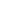and a base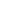, so that the base is a primitive root modulo the modulus.
Alice and Bob each choose a secret keyandrespectively – ideally, randomly generated. The parties then exchange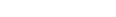(for Alice) and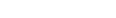(for Bob).
Alice now has received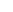. She goes on to compute the shared secretby calculating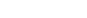and Bob computes it by calculating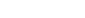.
The whole story is premised on the equality of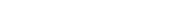That this holds nearly trivially true should be evident from substituting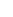forand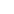for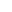. Then,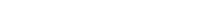Thus, both parties get the same shared secret. An eavesdropper would be able to getand. Given a sufficiently large prime for, in the range of 6-700 digits, the discrete logarithm problem of retrievingfromin the knowledge ofandis not efficiently solvable, not even given fairly extensive computing resources.

# Let’s get coding!

## Ingredients

Okay, time to get coding! We’ll be coding a brief demonstration of how this algorithm can be worked out in Python. You can find the fully developed example as a Github Gist. We’ll need three things for this to work:

1. A safe random number generator. You could do worse than the byte random function in the `ssl` library (`ssl.RAND_bytes`) or the `OpenSSL` library’s `OpenSSL.rand.bytes`.
2. Something to do SHA-256 hashing for us, as the key in the Diffie-Hellman exchange is not actually the shared secret but the SHA-256 hash thereof. A decent one for that `hashlib`.
3. We need to have the shared primes, and have an agreement on both the shared prime and the corresponding base (generator) – theand thein our scheme. RFC 3526 lists a few canonical primes known as numbered groups, with bit lengths from 1536 to 8192. For ultimate paranoia, we’ll be using Group 18, with generator 2 and 8192 bits.
4. Finally, we need to have an object that will handle state for us. We’ll start by creating the state holder.

## Imports and other stuff

As said, we’ll need something to handle hashing for us. In addition, we will need a random generator. We’ll be going with the one in the `SSL` library.

```import hashlib
import ssl
random_function = ssl.RAND_bytes```

That solves our first two ingredients. Then, we’ll need to define the prime group we will be using as a constant. An expansive implementation would contain all groups, but for now, we’ll simply set the desired group as a constant.

```# Copy the prime from RFC 3526... hex representations are the most economical.
PRIME_18 = 0xFFFFFFFFFFFFFFFFC90FDAA2216...7160C980DD98EDD3DFFFFFFFFFFFFFFFFF
GENERATOR = 2```

## Creating the object

To hold state during the whole process, we’ll start by scaffolding an object:

```class DiffieHellmanKeyExchange:
def __init__(self, key_length=650):
pass
# We'll need a good random generator to generate the private key...
def generate_private_key(self, length):
pass
# ...get the secret from own private key and the other party's public key...
def generate_secret(self, private_key, their_public_key):
pass
# ...and we'll need to generate a key from the secret by hashing it.
def generate_key(self, their_public_key):
pass```

## Key generation

First, we’ll need to create a random generator that gets us a random number of a given length, which we’ll use as our private key.

```def generate_private_key(self,  length):
_rand = 0
_bytes = length // 8 + 8
while(_rand.bit_length() < length):
_rand = int.from_bytes(random_function(_bytes), byteorder='big')```

What are we doing here? Most of this code deals with the fact that the random number generator thinks in bytes whereas we think in integers. As such, first, we’ll need to figure out the byte length of a number of a given length. Then, we’re using the `int.from_bytes()`  conversion function to give us the output of the `random_function()`  in an integer form, in big endian byte order (the `byteorder='big'` parameter specifies the endianness).
Generating the private key from this is simply generating a random number of a given length. The public key is a little more complex. We know that the public key is defined as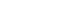whereis the generator (in our case, 2),is the private key generated in the previous step. Finally,is the prime group. It’s probably a good idea to frontload this into the creation of the object. As such, let’s go back to our object and rejazz our `__init__`. And while we’re at it, we should make sure users generate useful keys – in other words, let’s keep users from creating any keys less than 600 digits long.

```class DiffieHellmanKeyExchange:
def __init__(self, key_length=600):
self.key_length = max(600, key_length)
self.prime = PRIME_18
self.generator = GENERATOR
self.private_key = self.generate_private_key(self.key_length)
self.public_key = pow(self.generator, self.private_key, self.prime)```

This creates a self-catering class that creates its own keys upon initialisation, which is fairly handy to have.

## Generating the secret

To obtain the shared secret, we’ll need to calculatefor Alice andfor Bob. We’ll be feeding this function the other party’s public key:

```def generate_secret(public_key):
self.shared_secret = pow(public_key, self.private_key, self.prime)```

Finally, let’s bolt on the hashing code to get the key:

```shared_secret_bytes = self.shared_secret.to_bytes(self.shared_secret.bit_length // 8 + 1, byteorder = 'big')
hash_alg = hashlib.sha256()
self.key = hasher.update(bytes(shared_secret_bytes)).digest()```

Once again, we’ll need to deal with the fact that the hash algorithm needs an array of bytes lest hashlib’s SHA-256 won’t be able to hash it.

## The finished product

```import hashlib
import ssl
PRIME_18 = 0xFFFFFFFFFFFFFFFFC90FD...
GENERATOR = 2
class DiffieHellmanKeyExchange:
def __init__(self, key_length=600):
self.key_length = max(600, key_length)
self.prime = PRIME_18
self.generator = GENERATOR
def generate_private_key(self, length):
_rand = 0
_bytes = length // 8 + 8
while(_rand.bit_length() < length):
_rand = int.from_bytes(random_function(_bytes), byteorder='big')
self.private_key = _rand
def generate_public_key(self):
self.public_key = pow(self.generator, self.private_key, self.prime)
def generate_secret(self, public_key):
self.shared_secret = pow(public_key, self.private_key, self.prime)
shared_secret_bytes = self.shared_secret.to_bytes(self.shared_secret.bit_length() // 8 + 1, byteorder='big')
hash_alg = hashlib.sha256()
hash_alg.update(bytes(shared_secret_bytes))
self.key = hash_alg.hexdigest()
```

By far not the smoothest way to do it, but we have created a fully functional key exchange system from, basically, scraps – a hash generator and a random number generator – in all of 24 lines of code. Not bad.

## Does it work???

Probably, and here’s how you can test it:

```if __name__ == '__main__':
a = DiffieHellmanKeyExchange()
b = DiffieHellmanKeyExchange()
a.generate_private_key(1024)
b.generate_private_key(1024)
a.generate_public_key()
b.generate_public_key()
a.generate_secret(b.public_key)
b.generate_secret(a.public_key)
if a.key == b.key:
print("It works!")
else:
print("Ooooops...")```

If all is well, the console ought to let you know that it indeed works.

# The moral of the story

Other than Python’s awesome expressiveness (to be fair, most modern languages let you implement this in the same number of lines, roughly!), a big question is what’s so cool about this. The answer is that Diffie-Hellman is not only an extremely elegant solution to a complex problem, it’s also a fantastic example of an every-day algorithm anyone with basic mathematical knowledge can not only understand but actually build and manipulate. As a society in which cryptography is getting a bad rap (see the recent #UnlockJustice campaign), many prefer fear to knowledge and many more prefer ignorance to an understanding of how they, as citizens, can build and use the tools to protect their secrets from government overreach.
There is an increased interest in banning, or limiting the use, of cryptography software. The day may come when the only cryptography will be what we write ourselves, and it’s never been more important and more of a citizenship skill to understand and know how to implement basic cryptography primitives.

References

↑1 As a child, I once built a pseudorandom number generator from a sound card, a piece of wire and some stray radio electronics, which basically rested on a sampling of atmospheric noise. I was surprised to learn much later that this was the method the KGB used as well. Under pressure from the advancing German Wehrmacht in 1941, they had duplicated over 30,000 pages worth of OTP code. This broke the golden rule of OTPs of never, ever reusing code, and ended up with a backdoor that two of the most eminent female cryptanalysts of the 20th, Genevieve Grotjan Feinstein and Meredith Gardner, on whose shoulders the success of the Venona project rested, could exploit. It deserves noting that the D-H key exchange algorithm was another of those inventions that were invented twice but published once. In 1975, the GCHQ team around Clifford Cocks invented the same algorithm, but was barred from publishing it. Their achievements weren’t recognised until 1997. I know someone who has Group 14 tattooed on the sole of his foot. Why? No reason, I’m told.I'm a data scientist and computational epidemiologist focusing on the intersection of public health, data science and artificial intelligence.